Function Repository Resource:

# GrayCodeSubsetIndex

Find the index of a subset in Gray code order

Contributed by: Wolfram Staff (original content by Sriram V. Pemmaraju and Steven S. Skiena)
 ResourceFunction["GrayCodeSubsetIndex"][list,s] gives the zero-based index of the subset s in the Gray code ordering of the subsets of list.

## Details and Options

In Gray code order, subsets differ from their neighbors by the insertion or deletion of one element.
The index of the empty set is 0.

## Examples

### Basic Examples (1)

The index of a subset in Gray code order:

 In:=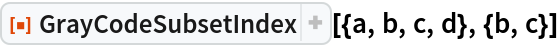Out=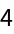### Properties and Relations (2)

Define a List:

 In:=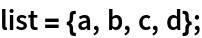Use the resource function GrayCodeSubsets to get the subsets of the List in Gray code order:

 In:=Out=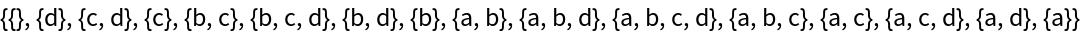Use GrayCodeSubsetIndex to find the index of each subset:

 In:=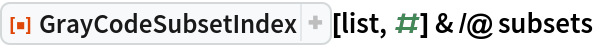Out=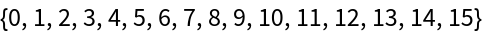Positions are offset by one:

 In:=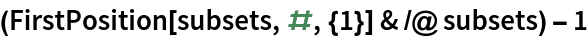Out=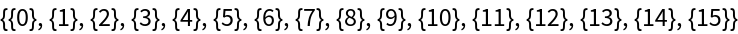Get the subsets in Gray code order sequentially using the resource function NthGrayCodeSubset:

 In:=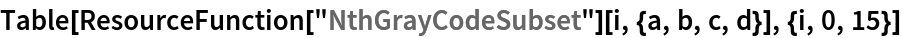Out=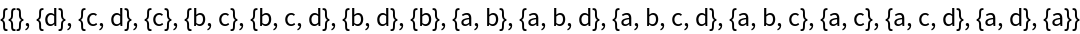Their respective indices:

 In:=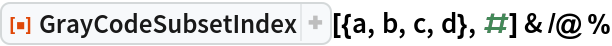Out=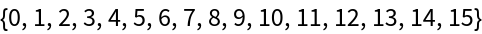## Version History

• 1.0.0 – 24 July 2020